# Loops Repetition Statements Repetition Statements Repetition statements allow

• Slides: 23Loops Repetition Statements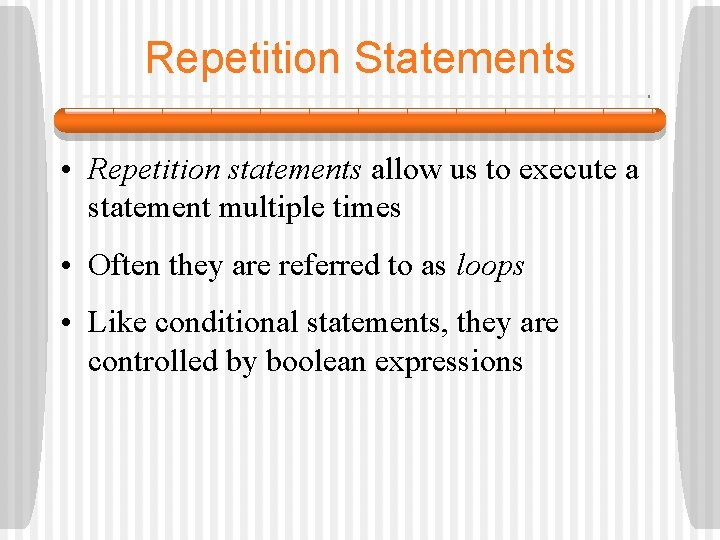Repetition Statements • Repetition statements allow us to execute a statement multiple times • Often they are referred to as loops • Like conditional statements, they are controlled by boolean expressions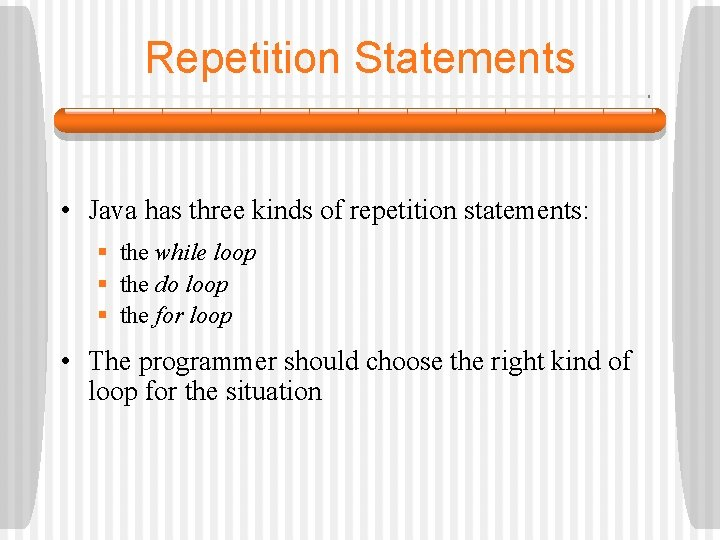Repetition Statements • Java has three kinds of repetition statements: § the while loop § the do loop § the for loop • The programmer should choose the right kind of loop for the situation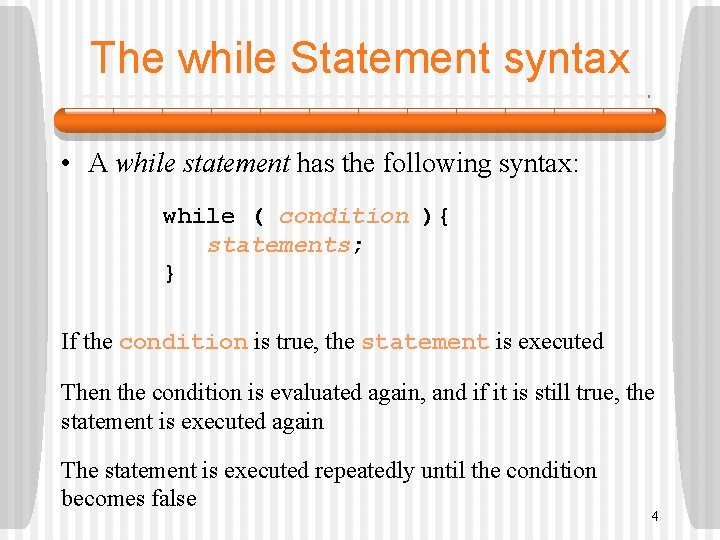The while Statement syntax • A while statement has the following syntax: while ( condition ){ statements; } If the condition is true, the statement is executed Then the condition is evaluated again, and if it is still true, the statement is executed again The statement is executed repeatedly until the condition becomes false 4Logic of a while Loop condition evaluated true statement falseExample 1: • An example of a while statement: int count = 1; while (count <= 3){ System. out. println (count); count++; }Example 2 • An example of a while statement: int count = 1; while (count < 2){ System. out. println (count); count++; }Example 3 • An example of a while statement: int count = 7; while (count < 7){ System. out. println (count); count++; }Example 4 • An example of a while statement: int count = 2; while (count < 3){ System. out. println (count); }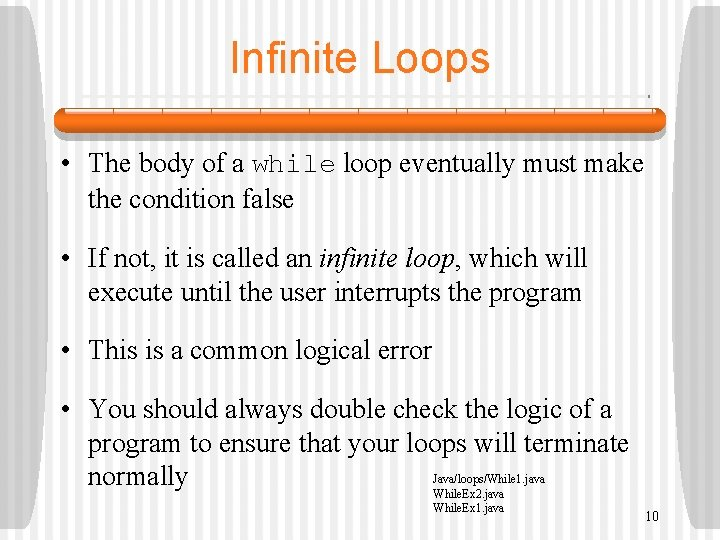Infinite Loops • The body of a while loop eventually must make the condition false • If not, it is called an infinite loop, which will execute until the user interrupts the program • This is a common logical error • You should always double check the logic of a program to ensure that your loops will terminate Java/loops/While 1. java normally While. Ex 2. java While. Ex 1. java 10Nested Loops • Similar to nested if statements, loops can be nested as well • That is, the body of a loop can contain another loop • For each iteration of the outer loop, the inner loop iterates completely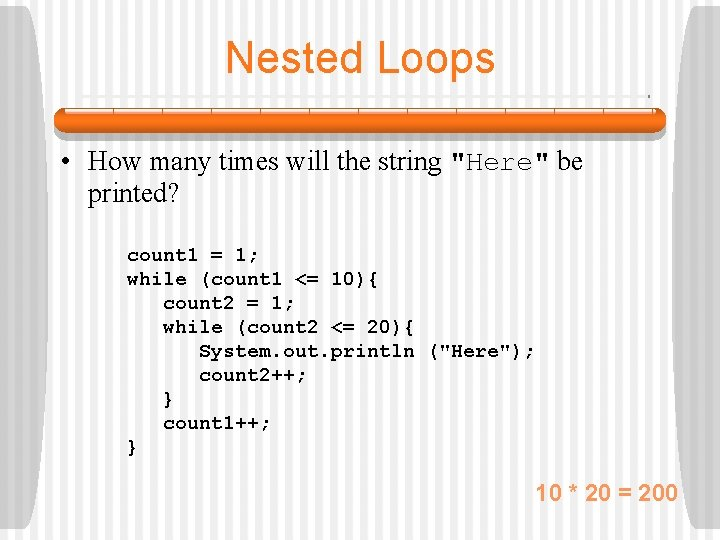Nested Loops • How many times will the string "Here" be printed? count 1 = 1; while (count 1 <= 10){ count 2 = 1; while (count 2 <= 20){ System. out. println ("Here"); count 2++; } count 1++; } 10 * 20 = 200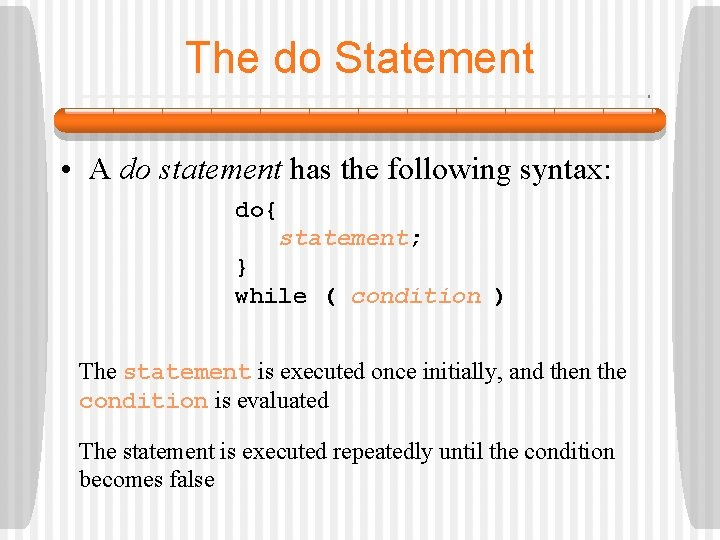The do Statement • A do statement has the following syntax: do{ statement; } while ( condition ) The statement is executed once initially, and then the condition is evaluated The statement is executed repeatedly until the condition becomes false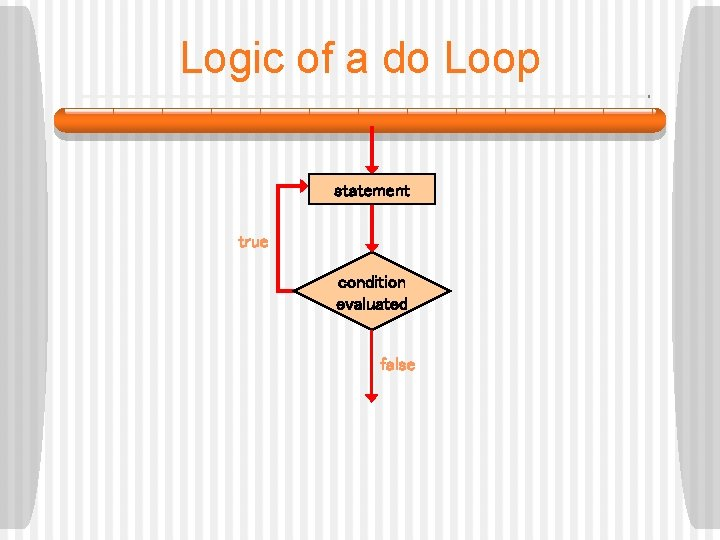Logic of a do Loop statement true condition evaluated falseThe do Statement • An example of a do loop: int count = 0; do{ count++; System. out. println (count); } while (count < 3);The do Statement • An example of a do loop: int count = 100; do{ count++; System. out. println (count); } while (count < 3); The body of a do loop executes at least onceComparing while and do The while Loop The do Loop statement condition evaluated true statement true false condition evaluated falseThe for Statement • A for statement has the following syntax: The initialization is executed once before the loop begins The statement is executed until the condition becomes false for ( initialization ; condition ; update ){ statements; } The increment portion is executed at the end of each iterationLogic of a for loop initialization condition evaluated true statements update falseThe for Statement • A for loop is functionally equivalent to the following while loop structure: initialization; while ( condition ){ statement; update; }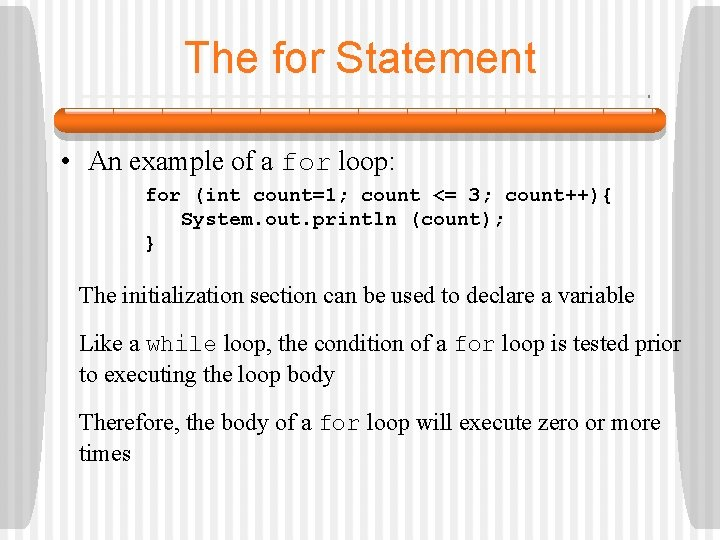The for Statement • An example of a for loop: for (int count=1; count <= 3; count++){ System. out. println (count); } The initialization section can be used to declare a variable Like a while loop, the condition of a for loop is tested prior to executing the loop body Therefore, the body of a for loop will execute zero or more timesThe for Statement • Each expression in the header of a for loop is optional • If the initialization is left out, no initialization is performed • If the condition is left out, it is always considered to be true, and therefore creates an infinite loop • If the increment is left out, no increment operation is performed Java/Loops. For 1. java For 2. java, For 3. java, For 4. java,Questions? ? ? Java/Loops/For. While. java For. Inside. While. java